# 五年级奥数和倍问题试卷及答案一、填空题

1.小红和妈妈的年龄加在一起是40岁，妈妈年龄是小红年龄的4倍，小红有

__________岁，妈妈有 __________岁。

2.生产队养公鸡、母鸡共404只，其中公鸡是母鸡的3倍，公鸡养了__________只，母鸡养了__________只。

3.小明买大单和小单线共25本，其中大单线的本数比小单线的本数的2倍多4本，大单线的本数有__________本，小单线的本数有 __________本。

4.师傅和徒弟共生产零件190个，师傅生产的个数比徒弟的3倍少10个;师、徒各生产 __________个？

5.A、B两人同时从学校出发相背而行，2小时共行48千米，A的速度是B的2倍，A的速度是每小时 __________ 千米，B的速度是每小时__________ 千米.

6.一块长方形木板，长是宽的2倍，周长是54厘米.这个长方形木板的面积是 _________平方厘米。

7.甲乙两个冷藏库原来共存肉92吨，从甲库运出28吨后，乙库存肉比甲库的4倍少6吨，甲库原来存肉__________吨，乙库原来存肉__________吨。

8.两个粮仓共存粮2200千克，由乙仓运出210千克，甲仓存的粮食是乙仓的2倍少380千克，甲仓库原来存粮食__________千克，乙仓库原来存粮食__________千克。

9.小红有30支铅笔，小兰有45支铅笔，小兰给小红__________支后，小红的支数是小兰的2倍。

10.姐姐有320元钱，弟弟有180元钱，弟弟给姐姐__________钱后，姐姐的钱比弟弟的钱多3倍？

二、解答题

11.甲乙粮仓共存粮1038吨，如果把甲仓存的粮食放到乙仓9吨，两仓库的粮食就一样多了，甲粮仓原来存粮食多少吨，乙粮仓原来存粮食多少吨？

12.两个数相除，商3余10，被除数，除数，商的和是163，被除数是多少，除数是多少？

13.小红铅笔的支数是小明的2倍，她从中拿出15支捐给了希望工程，正好是小红小明支数的总和的一半，小红原有铅笔多少支？

14.三个饲养场共养1600头牛，第二饲养场养牛的头数是第一饲养场的2倍，第三饲养场养的头数是第二饲养场的2倍多60头，三个饲养场各养牛多少头？

答案解析：

一、填空题

1.4+1=5  40÷5=8(岁)  8×4=32(岁)

答:小红的年龄是8岁,妈妈的年龄是32岁。

2.1+3=4      404÷4=101(只)     101×3=303(只)

答:有母鸡101只,公鸡303只。

3.25-4=21(本)      1+2=3     21÷3=7(本)    7×2+4=18(本)

答:买大双线7本,买大单线18本。

4.190+10=200(个)   1+3=4(倍)   200÷4=50(个)  50×3-10=140(个)

答:徒弟做50个,师傅做140个。

5.48÷2=24(千米)     1+2=3      24÷3=8(千米)    8×2=16(千米)

答:A的速度是每小时行16千米,B的速度是每小时行8千米。

6. 54÷2=27(厘米)1+2=3 27÷3=9(厘米)9×2=18(厘米)9×18=162(平方厘米)

答:长方形木板的面积是162平方厘米。

7.92-28+6=70(吨)1+4=5  70÷5=14(吨)14+28=42(吨)14×4-6=50(吨)

答:甲库原来存肉42吨,乙库原来存肉50吨。

8.2200-210+380=2370(公斤)1+2=32370÷3=790(公斤)

790+210=1000(公斤)790×2-380=1200(公斤)

答:甲仓存粮1200公斤,乙仓存粮1000公斤。

9.30+45=75(支)1+2=3   75÷3=25(支)   45-25=20(支)

答:小兰应给小红20支。

10.320+180=500(元)  1+1+3=5   500÷5=100(元) 180-100=80(元)

答:弟弟给姐姐80元后,姐姐的钱数比弟弟的钱数多3倍。

二、解答题

11.1038÷2=519(吨)519+9=528(吨)519-9=510(吨)

答:原来甲仓存粮528吨,乙仓存粮510吨。

12. 163-3-10=150  150-10=140   140÷(1+3)=35    35×3+10=115

答:被除数是115,除数是35。

13. 15×2=30(支)30÷(1+2)=10(支)10×2=20(支)

答:小红原有铅笔20支。

14.1600-60=1540(头)2×2+1+2=7  1540÷7=220(头) 220×2=440(头)

440×2+60=940(头)

答:第一饲养场养牛220头,第二饲养场养牛440头,第三饲养场养牛940头。您还可以通过手机、平板电脑等移动设备访问石家庄奥数网，升学路上有我们相伴。>>[点击查看]

•欢迎扫描二维码
关注奥数网微信
ID：aoshu_2003

•欢迎扫描二维码
关注中考网微信
ID：zhongkao_com

### 教育导航

1.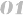北京站 上海站 广州站 深圳站
2.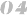天津站 武汉站 成都站 石家庄站
3.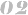南京站 杭州站 济南站 苏州站
4.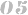郑州站 沈阳站 太原站 重庆站
5.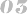长沙站 合肥站 宁波站 青岛站